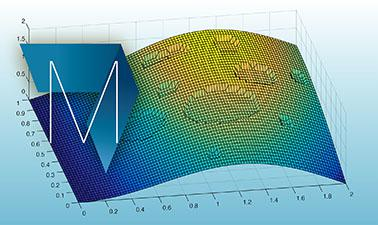# MATLAB and Octave for Beginners

Learn MATLAB and Octave and start experimenting with matrix manipulations, data visualizations, functions and mathematical computations.## There is one session available:

After a course session ends, it will be archived.
Estimated 4 weeks
5–6 hours per week
Self-paced

Take an exciting crash course in MATLAB and Octave programming. Both languages allow users to experiment with advanced mathematical functions and produce exciting matrix visualizations.

In this hands-on, self-paced introductory course, students will learn step by step how to use these mathematical tools to write functions, calculate vectors and matrices and plot graphical representations of results. Explore ways to organize your work using scripts and functions to improve productivity.

### At a glance

• Institution: EPFLx
• Subject: Computer Science
• Level: Introductory
• Prerequisites:

No background in programming needed. However, the following background in math concepts are necessary:

• functions of a variable
• graph of a function
• (optionally) function of two variables
• vector and matrix manipulation
• manipulation de vecteurs et matrices
• Language: English

# What you'll learn

Skip What you'll learn
• To use and to write scripts and functions using MATLAB and Octave
• To work with vectors and matrices
• To handle data files
• To plot graphics and save them in pdf and jpg formats
• To write interactive programs

# Learner testimonials

Skip Learner testimonials

"Thank you for this course and for using Octave. My compliments for this course. It has been really amazing how you have been able to expose the main topics of the Octave programming in hardly a month. The only troubles I have found are related with the quizzes, and I know that have been caused due some problems of Mathworks related with the use of Octave in this course. I am a hard defender of the free software, so I would like to encourage you to maintain this politics in despite of this kind of problems. It could be great helping to develop some automatic Octave system on the server side to do easy the task of examining. Great... and Thank You!"
- Previous Student